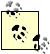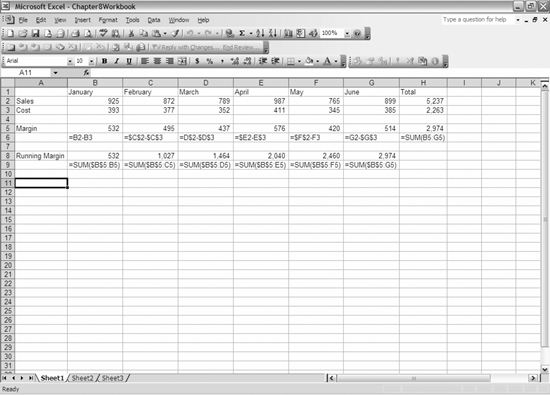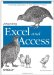# 8.1. Writing Flexible Formulas

 < Day Day Up >

In addition to dealing with the Excel objects, understanding how to use R1C1 format to write formulas makes coding much easier. For example, subtracting the cell one row above from the cell two rows above is very straightforward when using R1C1 notation . If you use A1 notation, you have to go through multiple steps to write the string. First, figure out what column and row you are in, and then turn the column number into a letter. Next, create strings by concatenating the column letter with the row numbers needed for your formula. Doing this for several columns would get tiresome, not to mention difficult to follow. You could also perform the calculation in VBA by using the row and column numbers with the Cells object, resulting in a fixed value instead of a formula. This is likely not what you want for most reporting because it can lead to inconsistent information if the original numbers are changed. Using the R1C1 format, you refer to the other cells by the number of rows or columns in relation to the current cell. In the previous example, the formula in R1C1 notation would be = R[-2]C - R[-1]C, which calculates the cell.Excel uses R1C1 notation to refer to a cell by the row and column numbers. For example, cell B10 is in column 2 and row 10; refer to this as R10C2 by using R1C1 notation. R1C1 notation also allows you to refer to a cell in relation to the current cell when writing a formula. Instead of placing a number for the row or column, you can place a number in brackets after R or C to move the specified number of columns or rows to refer to the cell you want. When you use a number for the row or column, Excel shows it as a fixed reference by using a \$ in the formula. You can also refer to the row and column with a fixed reference for one and a relative reference for the other. R2C[-1] would refer to the second row of the column to the left of the current cell.

When you write formulas, there are several properties that you can set to write the formula or value (see Table 8-1). This chapter covers Formula, FormulaR1C1, and FormulaArray, which provide most of the functionality that you need.

##### Table 8-1. Excel formula properties of the range object

Property

Description

Formula

Returns or sets a formula using A1-style notation

FormulaArray

Returns or sets an array formula using R1C1-style notation

FormulaLocal

Returns or sets a formula using A1-style notation in the language of the user

FormulaR1C1

Returns or sets a formula using R1C1-style notation

FormulaR1C1Local

Returns or sets a formula using R1C1-style notation in the language of the user

Value

Returns or sets a fixed value

Let's look at returning R1C1 formula values so that you can get a feel for how to write them. Specifically, you need to understand how to set fixed and relative references. Look at the formulas in Figure 8-1, shown in A1-style notation below each result. Create a workbook similar to this one and press Alt+F11 to go to the Visual Basic Editor. Then, press Ctrl-G to go to Immediate Window.

The first formula to look at is cell B5, also shown as text in cell B6 in Figure 8-1. In this formula, use relative references so that if you copy them from left to right, they update to the correct formula. If you copy them down, this formula will not yield the correct result. Type the following line in the immediate window, and it shows you how to write this formula in R1C1-style notation:

`     ? sheets("Sheet1").Range("B5").FormulaR1C1`

The result comes back =R[-3]C-R[-2]C for the A1-style formula of =B2-B3. This R1C1-style formula works in any of the cells in row 5. Breaking down this formula, it tells Excel to subtract the cell two rows up in the current column from the cell three rows

##### Figure 8-1. The worksheet showing similar formulas written a variety of different waysup in the current column. Next, look at the formula in cell C5. Type the following line in the immediate window:

`     ? sheets("Sheet1").Range("C5").FormulaR1C1`

This formula result comes back =R2C3-R3C3 for the A1-style formula of =\$C\$2-\$C\$3. For this formula, the exact same A1-style formula result comes back regardless of the cell being because the formula tells Excel to subtract row 3 column 3 (C3) from row 2 column 3 (C2). When using R1C1 notation, whenever you use a row or column number and do not put it in brackets, it results in the row or column being preceded by a \$ in the formula. When a \$ is used in A1-style notation, Excel creates a fixed reference. If you copy a formula with fixed references, the portion with the \$ will not change, regardless of where you copy it. This is important to keep in mind as you decide how to write the formula. Type the following line in the immediate window:

`     ? sheets("Sheet1").Range("D5").FormulaR1C1`

The formula result comes back =R2C-R3C4 for the A1-style formula of =D\$2-\$D\$3. Notice that in this formula, dollar signs precede everything except the D in the first cell reference in the formula. Looking at the R1C1-style notation, you see that the column reference uses the current column C. If you put a number after the column reference, it returns the column with a dollar sign. Look at the others as well, but for the next formula, look at B8. Type the following line in the immediate window:

`     ? sheets("Sheet1").Range("B8").FormulaR1C1`

The formula results come back =SUM(R5C2:R[-3]C) for the A1-style formula of =SUM(\$B\$5:B5). This formula is important because same R1C1-style formula comes back for the entire row. This is the easiest way to write a running sum using a fixed reference for the beginning of the sum range and a relative reference for the end of the range. If you copy the formula across, it returns the correct formula for the running sum for each column.

Certainly look at the other formulas; looking at the resulting R1C1 formulas should help you write them yourself. I strongly encourage you to use this technique to write more complex formulas, since it is much easier to create the formula in the Excel GUI than to write it yourself.

 < Day Day Up >Integrating Excel and Access
ISBN: 0596009739
EAN: 2147483647
Year: 2005
Pages: 132

Similar book on Amazon